# Does Wien bridge oscillator have a closed-loop gain?

• genxium

## Homework Statement

By Wien bridge oscillator (abbreviated as WBO below) I refer to this circuit where the op-amp is assumed to be ideal and the output is the voltage drained from the upper end of R3.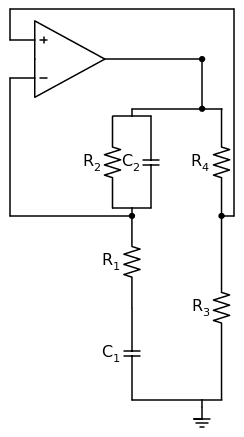What confuses me is that I hardly find a reference which explicitly states that a WBO has a closed-loop transfer function. By closed-loop transfer function I refer to that of a negative feedback block diagram as follows in s-domain, e.g. $ClosedLoopTransferFunc = \frac{G(s)}{1 - G(s)H(s)}$, if the WBO could be modeled like so.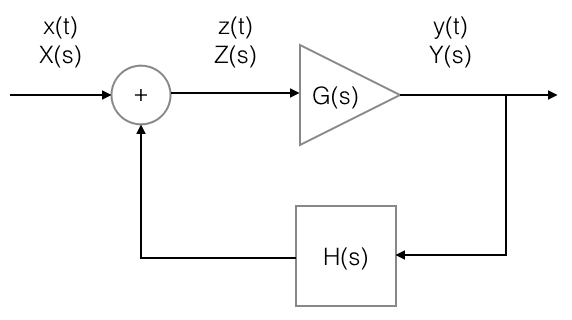## Homework Equations

Will be shown in the following section.

## The Attempt at a Solution

The difficult part for me is to define/find the input to the WBO. As told to me by an esteemed person in a personal email, the WBO has no input, and thus its closed-loop transfer function shouldn't be defined. However this is kind of weird to me. I understand that in terms of energy conservation the energy is drained from a DC pull and converted to sinusoidal waves and possibly heat, that's reasonable, but the magical word "convert" is so outstanding here.

What I think is that there should be an abrupt impulse, or sharp edge of voltage/current amplitude change when the oscillator is "switched on", and that impulse contains all kinds of frequency components to be amplified or attenuated w.r.t. individual closed-loop transfer function of each.

My attempt to quantify this intuition, especially how signals are "addible in s-domain", is to assume that in time-domain there's an ideal impulse which is proportional to a Dirac delta $\delta(t)$ as input signal $x(t)$ when "switched on" in the block diagram. Then $x(t)$ adds with the feedback signal $\mathcal{L}^{−1}\{H·Y\}(t)$ both at $t=0$ and after $t>0$ in time-domain, where $\mathcal{L}^{−1}$ is the inverse Laplace transform, $x(t \ne 0)=0$ and $\mathcal{L}^{−1}\{H·Y\}(t=0)=0$. Thus $X(s)$ and $H(s)$ adds in the s-domain as well due to the linearity of Laplace transform.

Moreover, my attempt to relate the 2 figures above is like the following.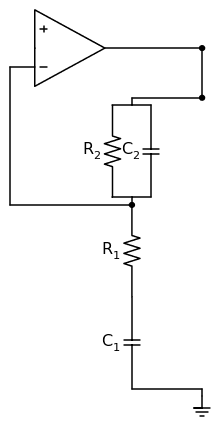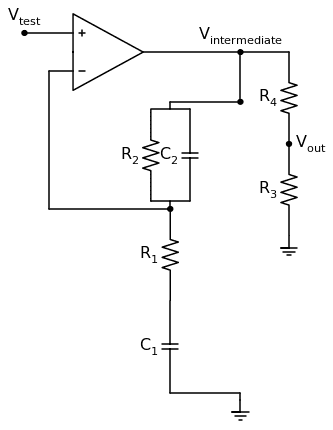where
• the first figure shows the ideal op-amp together with its negative feedback path being a finite-gain amplifier contributing to $G(s)$;
• the second figure shows how the "closed-loop", if exists, is opened, thus $H(s) = \frac{R_3}{R_3 + R_4}$.
Is my attempt problematic? If so, does the WBO have any well defined closed-loop transfer function by any means?

The condition for oscillation is that there's a frequency at which the voltage at the + input equals that at the – input. In practice, a gain slightly exceeding the minimum is set to ensure startup. In cases where spectral purity is desired, a means for automatically reducing the gain to the minimum necessary can be implemented.

Have you looked at the WikiP entry for Wien Bridge Osc which has a section ("Analysis") discussing closed loop gain and transfer function?

As a non-expert personal opinion, I'd have said : oscillators, when they have reached a stable amplitude, must have a closed loop gain of 1 at the operating frequency and <1 at all other frequencies. But they may be non-linear systems at this point. I would have thought, the aim of the stabilisation circuit is to keep the closed loop gain at 1 (at fo) while keeping the system as linear as possible.

As a non-expert personal opinion, I'd have said : oscillators, when they have reached a stable amplitude, must have a closed loop gain of 1 at the operating frequency and <1 at all other frequencies.

The loop gain (gain of the open loop) must be unity at nominal conditions.
In contrast, if we consider the oscillator as a "limiting case" of an amplifier (with a denominator approaching zero because of unity loop gain) the closed-loop gain approaches infinity (but a formula cannot be given).

Have you looked at the WikiP entry for Wien Bridge Osc which has a section ("Analysis") discussing closed loop gain and transfer function?

As a non-expert personal opinion, I'd have said : oscillators, when they have reached a stable amplitude, must have a closed loop gain of 1 at the operating frequency and <1 at all other frequencies. But they may be non-linear systems at this point. I would have thought, the aim of the stabilisation circuit is to keep the closed loop gain at 1 (at fo) while keeping the system as linear as possible.

Yes I read the Wikipedia page, and it uses a slightly different (yet classical) WBO structure for analysis. To my understanding the following information is given by that Wikipedia page.
• The first part of "Analysis" section refers to "loop gain" instead of "closed-loop transfer function" to figure out the proper value of Rb at stability. This part makes use of Barkhausen criteria implicitly by taking 1 - T(s) = 0 as the oscillation condition, the concept of "pole" doesn't come out.
• The second part of "Analysis" section is marked as "citation needed", and it's exactly where I started my search :)
There're mentions of the concept "pole" in this page, but according to my searches by far, they're actually "roots" of 1 - T(s) = 0 which comes from Barkhausen criteria w.r.t. the "loop gain" only. These "roots" would have been called "poles" if (1 - T(s)) were a denominator of a gain/transfer function. However I haven't found a proper way to find such function IF the input signal of a WBO is 0, e.g. the closed-loop transfer function could hardly be defined whilst no other transfer functions seem feasible.

The input signal to an oscillator is its output. So providing the loop gain is a tiny bit over unity then oscillations will build from noise. We rely on a tiny amount of nonlinearity in the gain to set a limit on the amplitude otherwise it would hit the supply rail.

## Homework Statement

By Wien bridge oscillator (abbreviated as WBO below) I refer to this circuit where the op-amp is assumed to be ideal and the output is the voltage drained from the upper end of R3.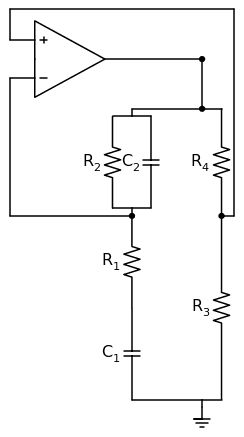Your block diagram is OK if you add a second feedback gain H2. H1 is - and H2 is +.
You can easily determine H1 and H2, then what is Y/X? In this picture, G = ∞.

Your block diagram is OK if you add a second feedback gain H2. H1 is - and H2 is +.
You can easily determine H1 and H2, then what is Y/X? In this picture, G = ∞.

@rude man I'm not sure whether I understand your argument, to my understanding, my block diagram belowis NOT PRESUMED TO BE a valid model of the Wien bridge oscillator below, and this is an essential point where I'm confused about. It was my fault that this wasn't clearly stated.

The reasons are as follows.
• The signals x(t), y(t) and z(t) are picked as VOLTAGES from different vertices in the Wien bridge oscillator. They're not marked in the figure because I'm still not sure about this argument.
• There's an ADDER in the block diagram which is not obviously and physically mapping to any component to the Wien bridge oscillator. As previously mentioned, I consider the signals x(t), y(t) and z(t) VOLTAGES, which don't simply add by joining wires to a vertex. This is why I proposed that an ideal Dirac Delta $x(t) = \delta (t)$ might be able to constitute this ADDER in a mathematical sense.

Moreover, by $G(s)$ I'd prefer to say that it corresponds to the following block INSTEAD OF the ideal op-amp alone, which might be referred to by you as $G = \infty$.

The following block contributes to $G(s) = \frac{(s^2 C_1 C_2 R_1 R_2 + s C_1 R_1 + s C_2 R_2 + s R_2 C_1 + 1)}{(s^2 C_1 C_2 R_1 R_2 + s C_1 R_1 + s C_2 R_2 + 1)}$. It's an s-domain dependent gain block which consists of the ideal op-amp and its negative feedback path.

Of course, one could make another blocking choice by taking the ideal op-amp with its positive feedback path together, but this is to my understanding a saturated configuration and thus doesn't yield a linear gain block. I'm open to the possibility that my blocking choice is wrong.As of the Barkhausen criterion, I personally DON'T take it as a sufficient condition to start up the oscillation at desired frequency. Here're my references for this argument.
I'm not saying that Barkhausen criterion is incorrect here, it is satisfied (at the desired frequency) in this case but just in general insufficient to guarantee a sinusoidal output.

However it's well known that closed-loop transfer function equals the Dirac delta response. Hence if the closed-loop transfer function were determined to be corresponding to a sinusoidal oscillation, e.g. $\frac{1}{s - j \cdot ( \omega_0)}$, it would have been kinda easier to imagine how Wien bridge oscillator wipes out noises after given an impulse input.

Last edited by a moderator:
Genxium, you forgot to write down the transfer function for the feedback block: H=R3/(R3+R4).
Because G(w=wo)=3/2 we require a nominal value H=2/3 for unity loop gain.

(Please, note that - for my opinion - the first of the two references you have given is incorrect as far as the comment on the Barkhausen criterion is concerned:"The Barkhausen Stability Criterion is simple, intuitive, and wrong ". It seems that the author did not understand the real content of this criterion, which is a necessary one.)

Your block diagram is OK if you add a second feedback gain H2. H1 is - and H2 is +.
You can easily determine H1 and H2, then what is Y/X? In this picture, G = ∞.

Yes - in principle, I agree. However, if we combine the gain block (G = ∞) with the negative feedback path (which is the frequency-dependent band-stop network), we arrive at a frequency dependent block G(s) as given in post#8. Hence, G(s) and H (fixed) form a positive feedback loop.

@rude man
The signals x(t), y(t) and z(t) are picked as VOLTAGES from different vertices in the Wien bridge oscillator. They're not marked in the figure because I'm still not sure about this argument.
• There's an ADDER in the block diagram which is not obviously and physically mapping to any component to the Wien bridge oscillator. As previously mentioned, I consider the signals x(t), y(t) and z(t) VOLTAGES, which don't simply add by joining wires to a vertex. This is why I proposed that an ideal Dirac Delta $x(t) = \delta (t)$ might be able to constitute this ADDER in a mathematical sense.
• The adder is impicit in the fact that the + and - inputs to the op amp constitute addition and subtraction of input signals. Everything is voltages, there is no need for confusion. You can map x, y and z of the block diagram to the schematic point-to-point as voltages.
I fail to see the idea of a delta function altogether. x(t) = δ(t) is dimensionaly incorrect as δ(t) has dimensions of 1/t.

The Barkausen criterion is necessary but not sufficient to provide a pure sinusoidal output. It's merely a reflection of the fact that the loop gain of an oscillator must be 1.0 (unity gain at zero phase shift), which in turn means that your y/x transfer function is infinite.. The nature of the output is determined by limiting aspects of the actual hardware. As someone else has pointed out, infinite gain means any tiny source of noise, always present, kicks the oscillator off.

Yes - in principle, I agree. However, if we combine the gain block (G = ∞) with the negative feedback path (which is the frequency-dependent band-stop network), we arrive at a frequency dependent block G(s) as given in post#8. Hence, G(s) and H (fixed) form a positive feedback loop.
? The required + feedback path R3/(R3 + R4) is absolutely missing in that diagram.
I'm not endorsing the idea of a block diagram for purposaes of analysis; we circuit types know better but that's what the OP seemed to be comfortable with, and it's totally OK.

? The required + feedback path R3/(R3 + R4) is absolutely missing in that diagram.
I'm not endorsing the idea of a block diagram for purposaes of analysis .

In my last answer I gave reference to post#8 - and the figure in post#8 clearly shows the pos. feedback path.
But - in general - I am with you regarding the value of a block diagram for analysis purposes.

In my last answer I gave reference to post#8 - and the figure in post#8 clearly shows the pos. feedback path.
But - in general - I am with you regarding the value of a block diagram for analysis purposes.
Depends on which figure. There are two of them. The first one the OP rejected. The second one has no + feedback.
Do we each get 10 points:Depends on which figure. There are two of them. The first one the OP rejected. The second one has no + feedback.
Do we each get 10 points:In any case - I suppose you know which figure I was referring to, dont you?
Question to genxium: Can you explain WHY the voltage divider must be connected to the + input?

In any case - I suppose you know which figure I was referring to, dont you?
Question to genxium: Can you explain WHY the voltage divider must be connected to the + input?

Is "voltage divider" referring to the path where $H(s) = \frac{R_3}{R_3 + R_4}$ is calculated from?

The voltage divider R3-R4 is connected to the + input.
My question was simply if you can explain WHY you have chosen this input (pos).
(Because for the original WIEN oscillator the resistive divider is connected to the - input).

The voltage divider R3-R4 is connected to the + input.
My question was simply if you can explain WHY you have chosen this input (pos).
(Because for the original WIEN oscillator the resistive divider is connected to the - input).

Understood. The Wien oscillator variant used here is not chosen for special purpose, it's by far just personal preference that the paths are "reversed" compared to the classical wbo.

However this question and @rude man 's comments make me think of a mistake I've been making. In a simulation software, the computer wouldn't slice a circuit into such simple block diagram and run its analysis like I did. To my understanding the transient simulation of lumped circuit is usually based on differential equations and boundary conditions of each component. Moreover, the "infinite" quantities, e.g. δ or Gideal op-amp in discussion, if being reasonable arguments, should find their proper roles in the simulation data -- assuming that modern simulation algorithms have good prediction ability.

I'll collect some data from SPICE simulation of the Wien oscillator, and see whether something new for me comes out.

Understood. The Wien oscillator variant used here is not chosen for special purpose, it's by far just personal preference that the paths are "reversed" compared to the classical wbo.
.
Just "personal preference"? Are you happy with such an answer?
OK - let me ask more specifically:
* We start with the original WIEN configuration (RC-bandpass in the pos. feedback loop).
* If we exchange the RC-series connection and the RC parallel block within the feedback path, we have a kind of bandstop network (with a transfer factor of 2/3 at the mid frequency where the phase is zero)..
* Hence, if we change the gain of the opamp amplfier from the previous value of "3" to the new value of 1.5=3/2 we can fulfill the oscillation condition bbecause of (2/3)*(3/2)=1. This requires an exchange of both gain determining resistors (R3, R4).
* This new circuit looks similar to your circuit - with one exception: The polarity of the opamps input nodes for the new circuit is reversed if compared with the oscillator circuit we are speaking of (as shown in your first post).
* NOW THE QUESTION AGAIN: Will both oscillators be able to oscillate (assuming, of course, correct dimensioning) ?

Just "personal preference"? Are you happy with such an answer?
OK - let me ask more specifically:
* We start with the original WIEN configuration (RC-bandpass in the pos. feedback loop).
* If we exchange the RC-series connection and the RC parallel block within the feedback path, we have a kind of bandstop network (with a transfer factor of 2/3 at the mid frequency where the phase is zero)..
* Hence, if we change the gain of the opamp amplfier from the previous value of "3" to the new value of 1.5=3/2 we can fulfill the oscillation condition bbecause of (2/3)*(3/2)=1. This requires an exchange of both gain determining resistors (R3, R4).
* This new circuit looks similar to your circuit - with one exception: The polarity of the opamps input nodes for the new circuit is reversed if compared with the oscillator circuit we are speaking of (as shown in your first post).
* NOW THE QUESTION AGAIN: Will both oscillators be able to oscillate (assuming, of course, correct dimensioning) ?

Apologize for bad wording, by "personal preference" I really meant "no satisfactory technical reason for the choice"Back to your question. I hope that you could confirm my understandings to the following.

By original or classical Wien oscillator, are you referring to this one?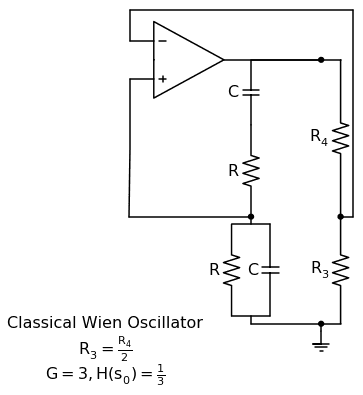And by the end of steps of modification, should I get this Wien oscillator variant?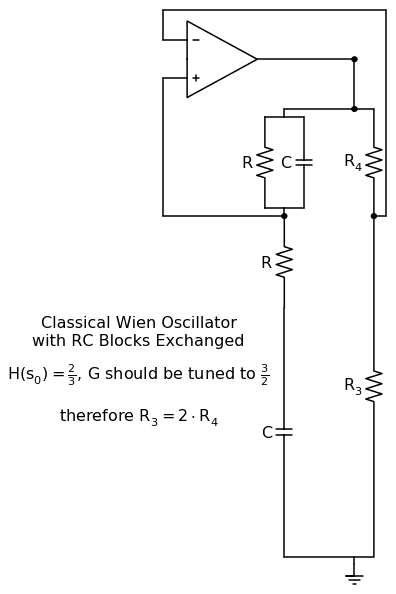If yes, I merely see that it's different from the one I used to start this thread/discussion, but haven't got an answer to your question yet. Will answer after some efforts on learning the software.

Yes - exactly. That was what I had in my mind.
Back to your first sentence: I really meant "no satisfactory technical reason for the choice.
But there is, indeed, a specific technical reason for your (first) choice. And the background of my question was if you are aware of this reason.
The answer (with explanation) to the question has a relation to the oscillation condition: Will every circuit with feedback oscillate if it meets the Barkhausen criterion?

genxium, do you have an answer - or are you really not interested to hear the answer?

genxium, do you have an answer - or are you really not interested to hear the answer?

Didn't mean to delay the reply but your modification to the classical WBO completely took down my guess on the input signal!

Unfortunately I haven't got an answer to your question yet but I want to probe more data by myself for a little while.

By using Ngspice and the netlist from here http://www.ecircuitcenter.com/Circuits/opwien/opwien.htm, it's verified that the modified WBO according to you doesn't oscillate (2 netlists enclosed) -- not even any noise showed up in the transient simulation. These facts are by far what I've mainly got.

#### Attachments

• wbo_spice.zip
1.5 KB · Views: 179
Didn't mean to delay the reply but your modification to the classical WBO completely took down my guess on the input signal!
.
Why do you again mention an input signal? Where and why? The whole circuit does not have (and does not need) any input signal!
By using Ngspice and the netlist from here http://www.ecircuitcenter.com/Circuits/opwien/opwien.htm, it's verified that the modified WBO according to you doesn't oscillate (2 netlists enclosed) -- not even any noise showed up in the transient simulation. These facts are by far what I've mainly got.
Yes - it is correct that the circuit does not oscillate. But - for a good understanding it is necessary to know the explanation rather than to rely upon simulation results only. Do you agree?

But - for a good understanding it is necessary to know the explanation rather than to rely upon simulation results only. Do you agree?

Of course! And I'm especially curiously about why it doesn't even start with any noise of noticeable amplitude -- sometime before I worked with several "incorrect" Hartley/Colpitts models which at least gave noises at the beginning during transient simulation. Not sure whether there's a configuration fault or instead a compliance with existing theories.

May I ask for a hint?

Of course! And I'm especially curiously about why it doesn't even start with any noise of noticeable amplitude -- sometime before I worked with several "incorrect" Hartley/Colpitts models which at least gave noises at the beginning during transient simulation. Not sure whether there's a configuration fault or instead a compliance with existing theories.
May I ask for a hint?

Each oscillator must comply with the oscillation criterion and it must have a fixed DC bias point within the linear transfer characteristics of the amplifier.
Are both conditions fulfilled?

Each oscillator must comply with the oscillation criterion and it must have a fixed DC bias point within the linear transfer characteristics of the amplifier.
Are both conditions fulfilled?

Understood.

Glad to say that the questions of this thread have all been resolved. I've put together involved netlist files on a git repository for reuse convenience https://github.com/genxium/WienBridgeOscTest.

May I add some more considerations?
It is true that the modification under consideration has no fixed and stable DC bias point and, hence, will not oscillate.
However, even the (novel) oscillation condition cannot be fulfilled:
The loop gain has - due to the reversed RC feedback path - now a band-stop characteristics (in comparison to the original WIEN network with a band-pass function). The resulting PHASE response of such a network has POSITIVE slope (rising phase). However, the updated (completed) oscillation criterion requires a NEGATIVE phase slope - see here:
https://www.researchgate.net/publication/255171263_On_a_rigorous_oscillation_condition?ev=prf_pub [Broken]

Last edited by a moderator: